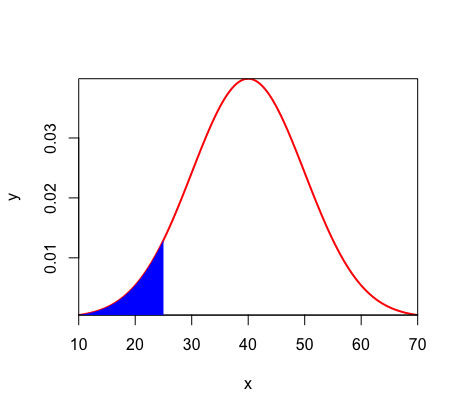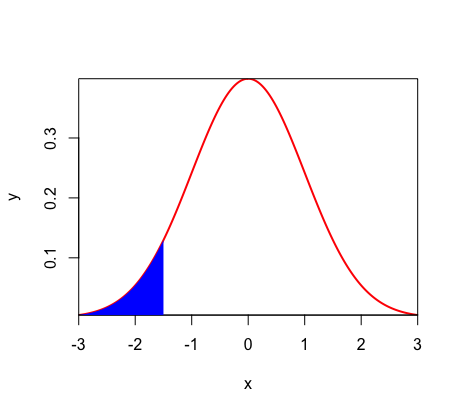How to Use the Normal Distribution & Z-Score to Find Probability

Density Curves

Recall that the normal distribution is a type of density curve, and that the area under a density curve is equal to the probability. Thus, we can use the area under a normal distribution to find probability.

*For more information on density curves, see the 'Density Curves' help page.

Standard Normal Density Curve

The standard normal density curve is centered at zero and has a standard deviation of one. In notation, this is expressed as x~N(0, 1).

On a standard normal density curve, each x-value will be equal to how many standard deviations x is above or below the mean. For example, on a standard normal density curve, the value x = 2 is 2 standard deviations above the mean because starting at 0, one must add two full standard deviations (i.e. 1 + 1) in order to reach x = 2.

Finding the Z-Score

Let's say you are given a normal distribution with mean 40 and standard deviation 10. You want to know the probability of getting 25 or less. This can also be expressed as P(x ≤ 25).The first step is to determine how far 25 is from the mean. If you start at 40 and go one standard deviation (i.e. 10) to the left, you get to 30. The next time, you cannot subtract another full standard deviation or else you would get 20. However, if you subtract half of a standard deviation (i.e. 5), you get 25. Thus, 25 is 1.5 standard deviations from the mean.

In the example above, we "standardized" the problem. By calculating how many standard deviations 25 is below the mean, we made it possible to plot this point on a standard normal density curve. Let's call this new point z = -1.5The value calculated above is known as the z-score.

The z-score tells you how many standard deviations x is above or below the mean.

Although we solved for the z-score using logic above, there is a more simple formula:

$$z = {{x - μ}\over σ}$$

If we had solved for the z-score using the formula instead of logic, it would have looked like this:

$$z = { {25-40}\over 10}$$  →  $$z = -1.5$$

Using the Z-Score to Find Probability

The original problem was to find the probability of getting 25 or less. Now that the x-value has been standardized and plotted on a standard normal density curve, all that remains is to find the area to the left of z = -1.5

There are two ways to accomplish this. One way is to look at a table of the standard normal distribution, which gives you certain values of z and the area below said value. If such a table is not provided, a TI-84 calculator can also be used.

Calculator Steps:

1) Hit '2nd' then 'VARS'

2) Hit 'normal cdf'

3a) FOR "LESS THAN"/AREA TO THE LEFT: (-999, z, 0, 1)*

* The first two numbers are the lower and upper bounds of the area in question where -999 (or some other arbitrary very negative number) is the lower bound and z is the upper bound. The second two numbers refer to the standard normal density curve where 0 is the mean and 1 is the standard deviation.

3b) FOR "GREATER THAN"/AREA TO THE RIGHT: (z, 999, 0, 1)

* The first two numbers are the lower and upper bounds of the area in question where z is the lower bound and 999 (or some other arbitrary very positive number) is the upper bound. The second two numbers refer to the standard normal density curve where 0 is the mean and 1 is the standard deviation.

After following these steps, the calculator computes an area of 0.0668. This means that the probability of getting 25 or less is 6.68%.